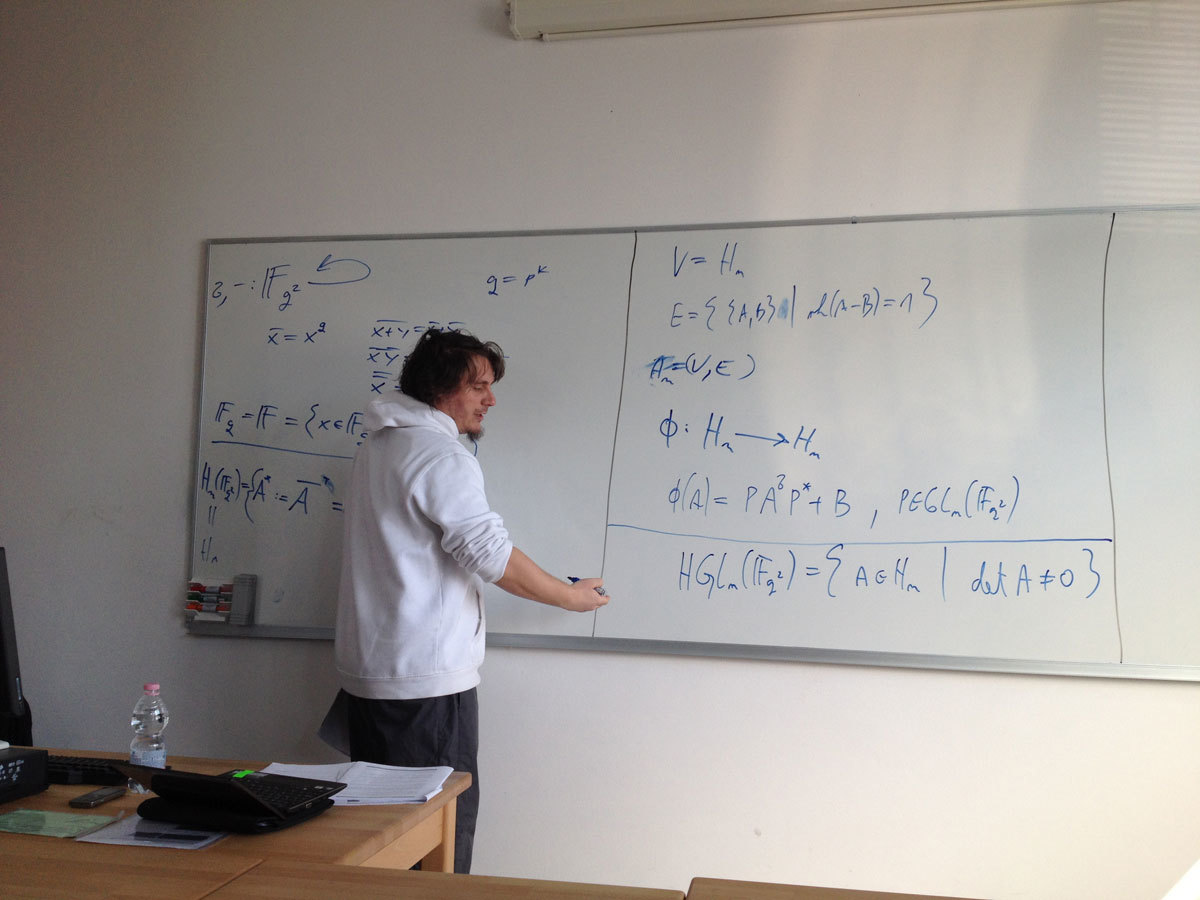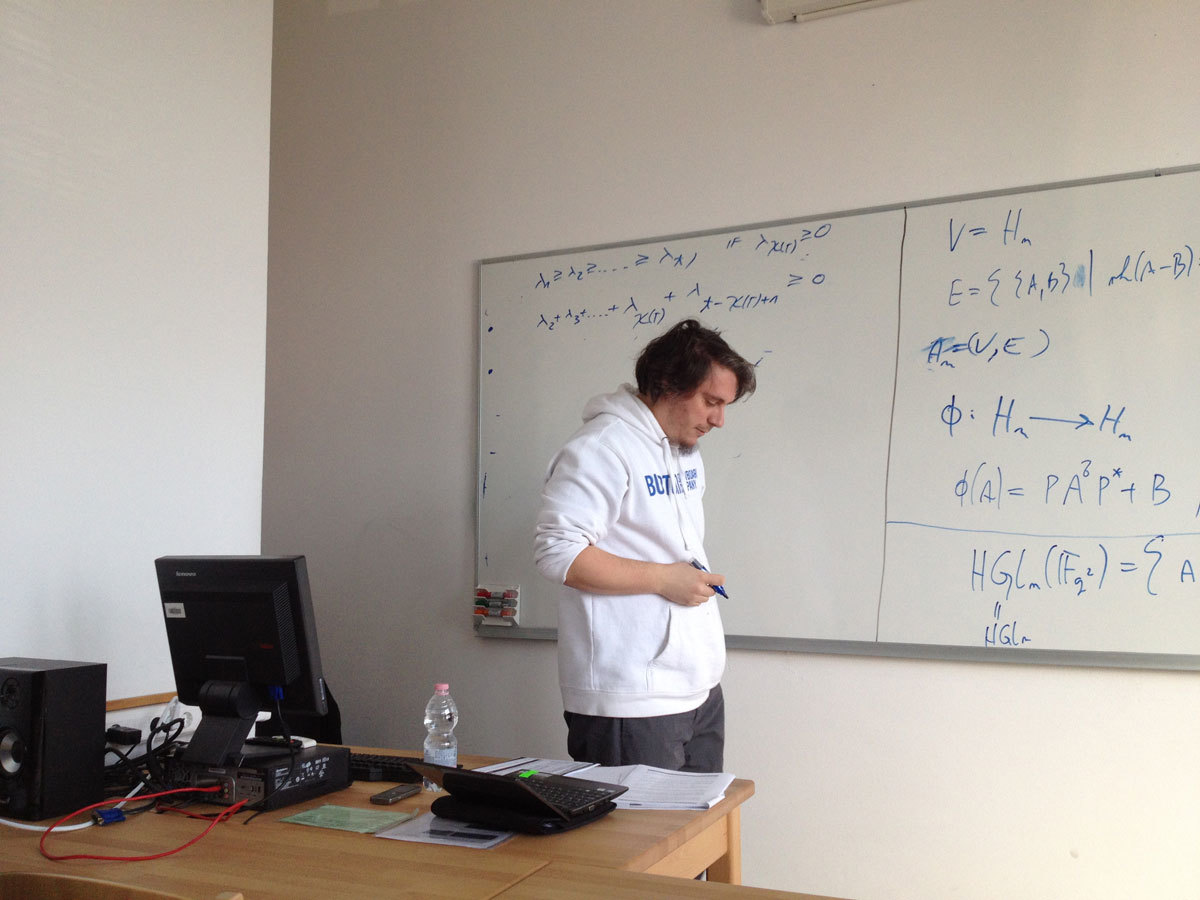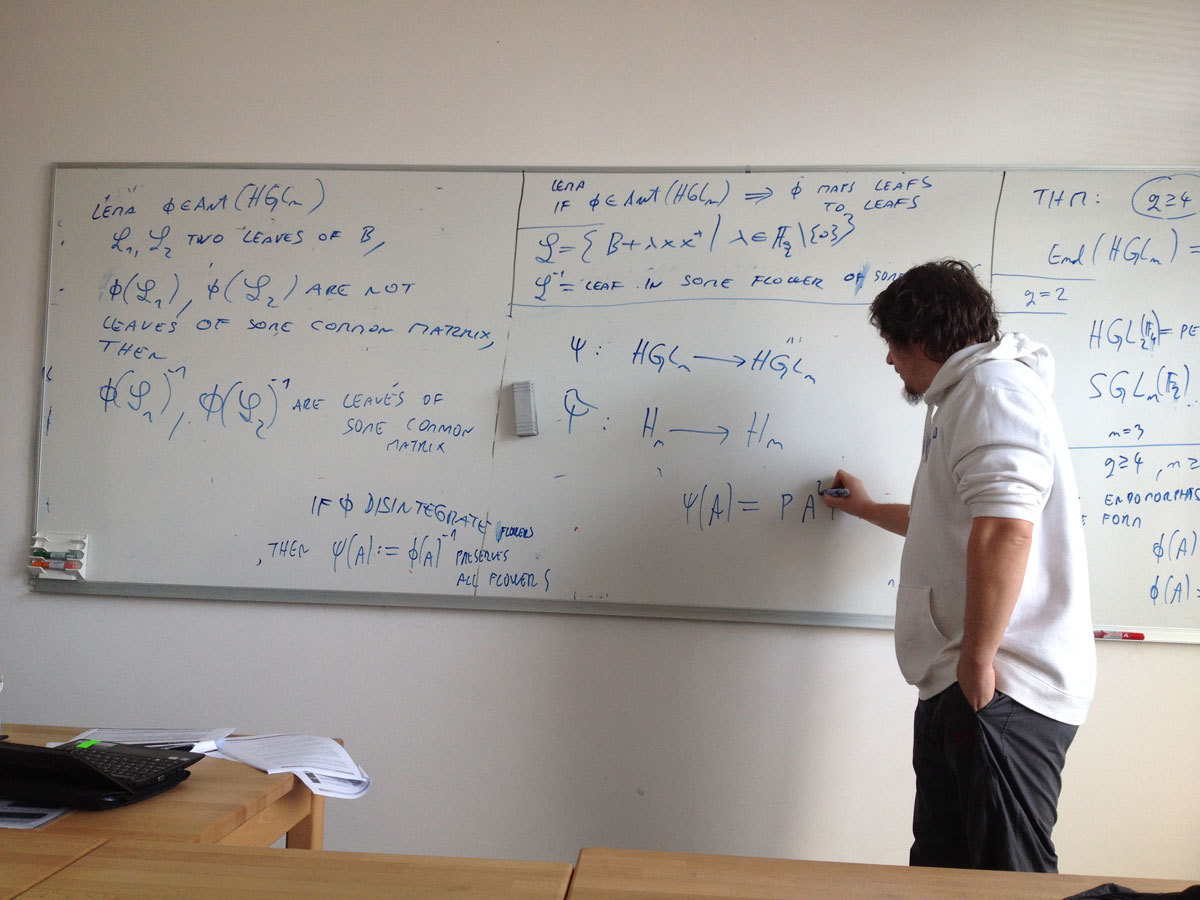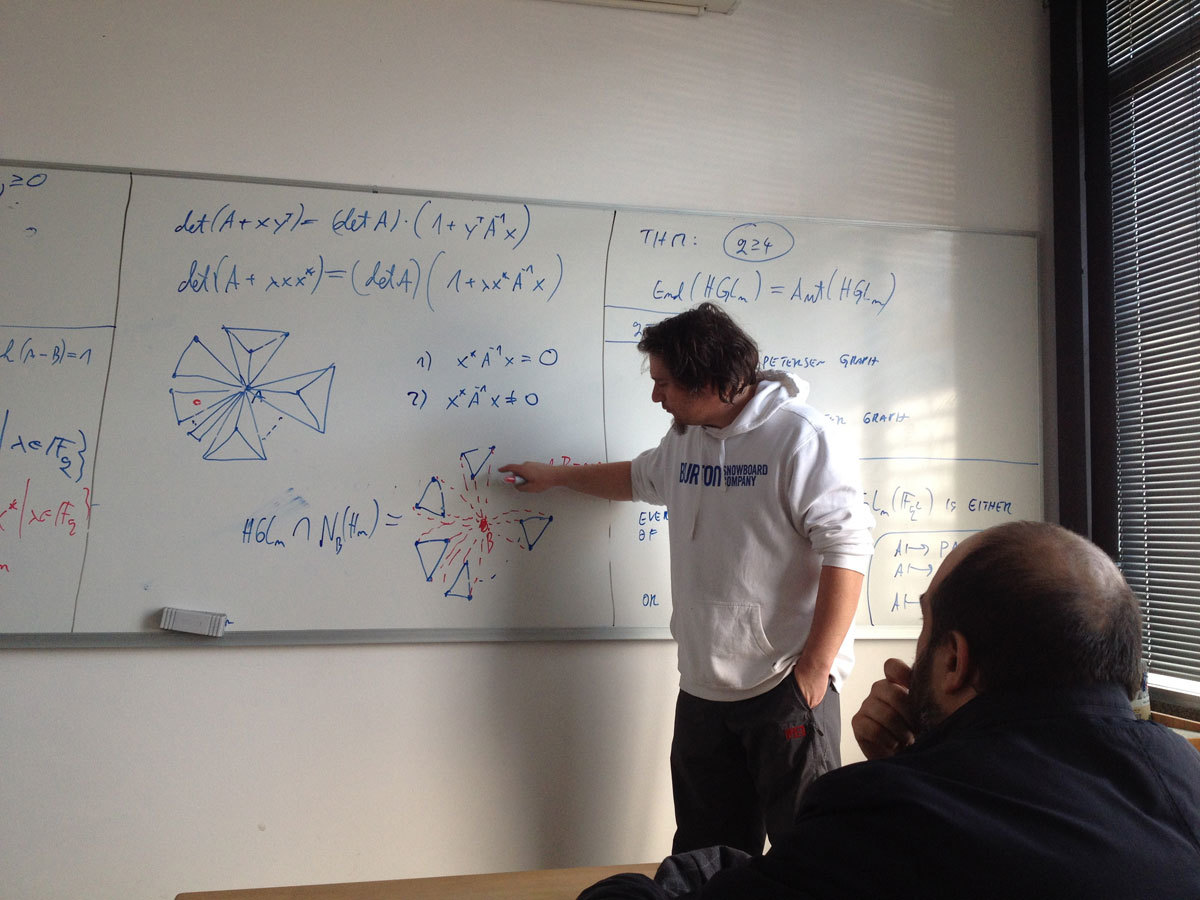Univerza na Primorskem Fakulteta za matematiko, naravoslovje in informacijske tehnologije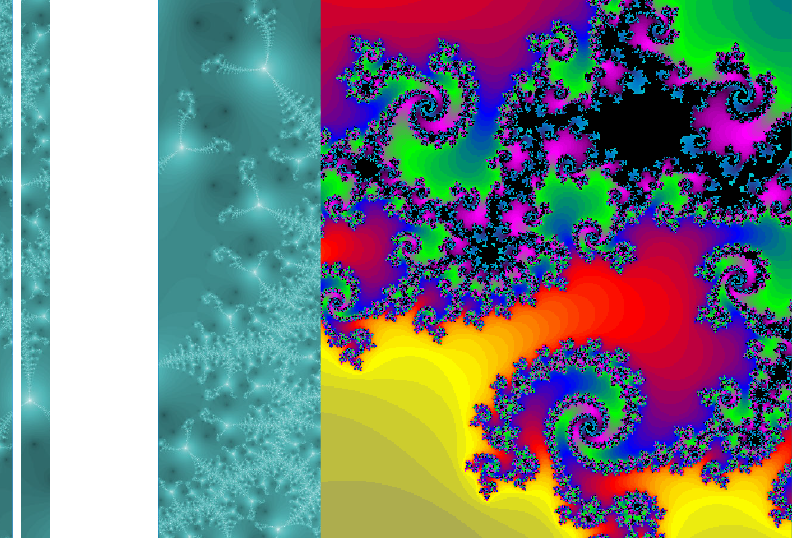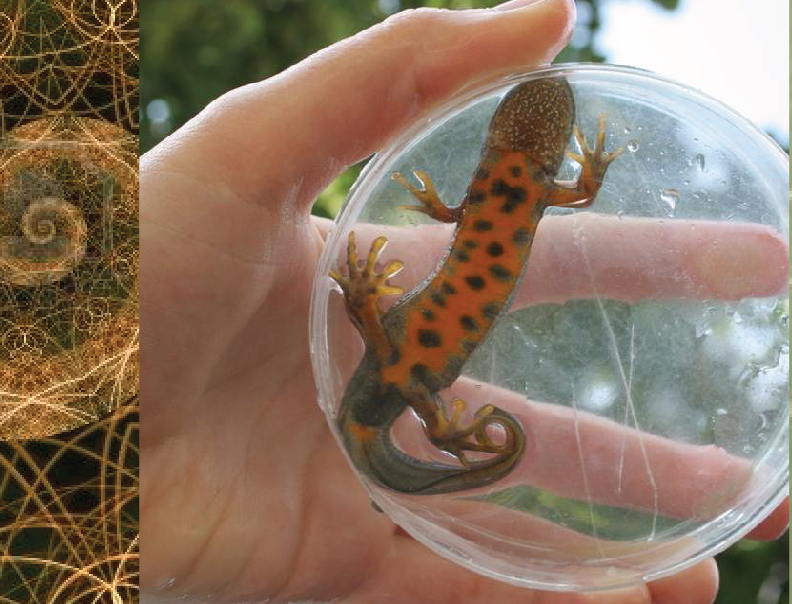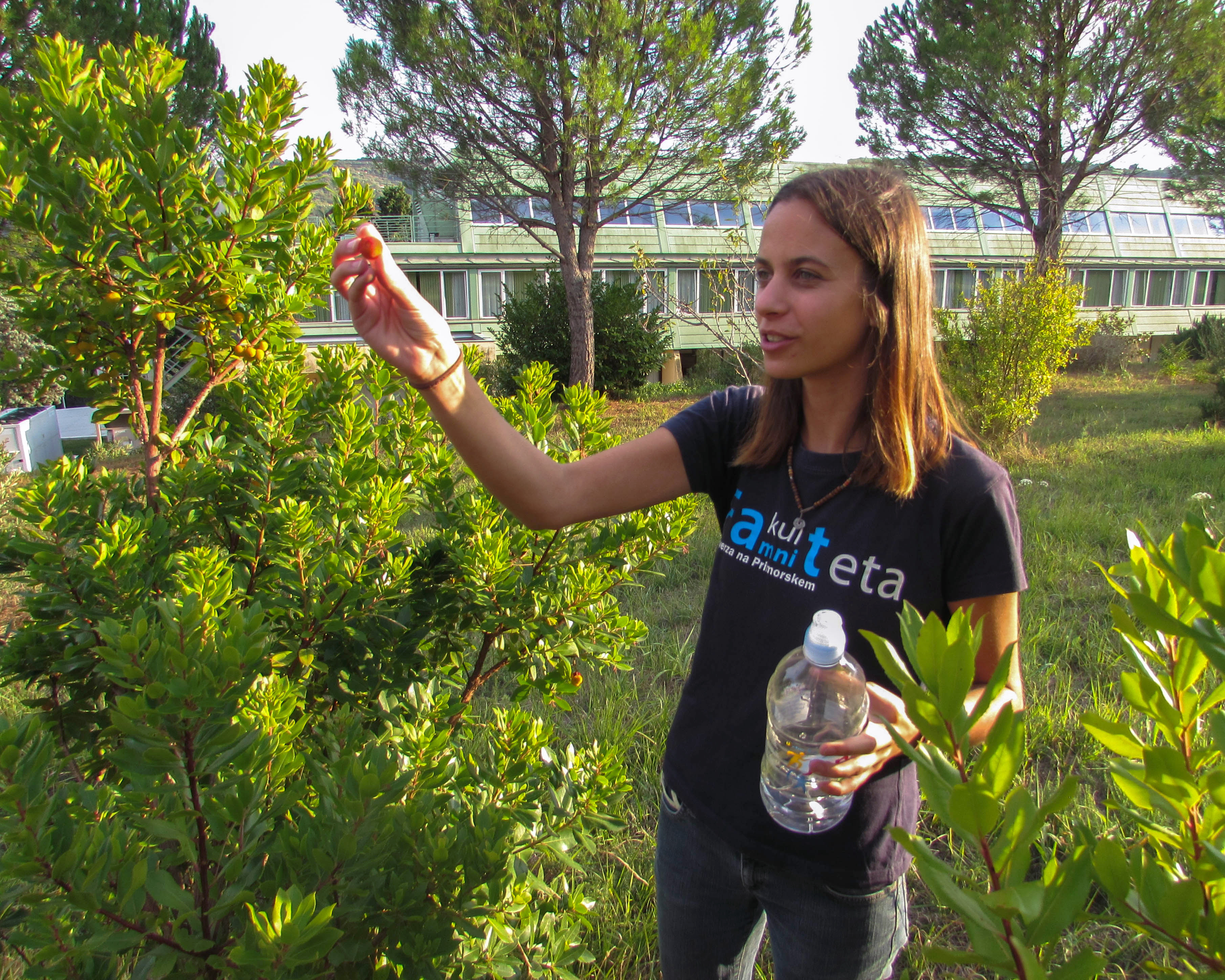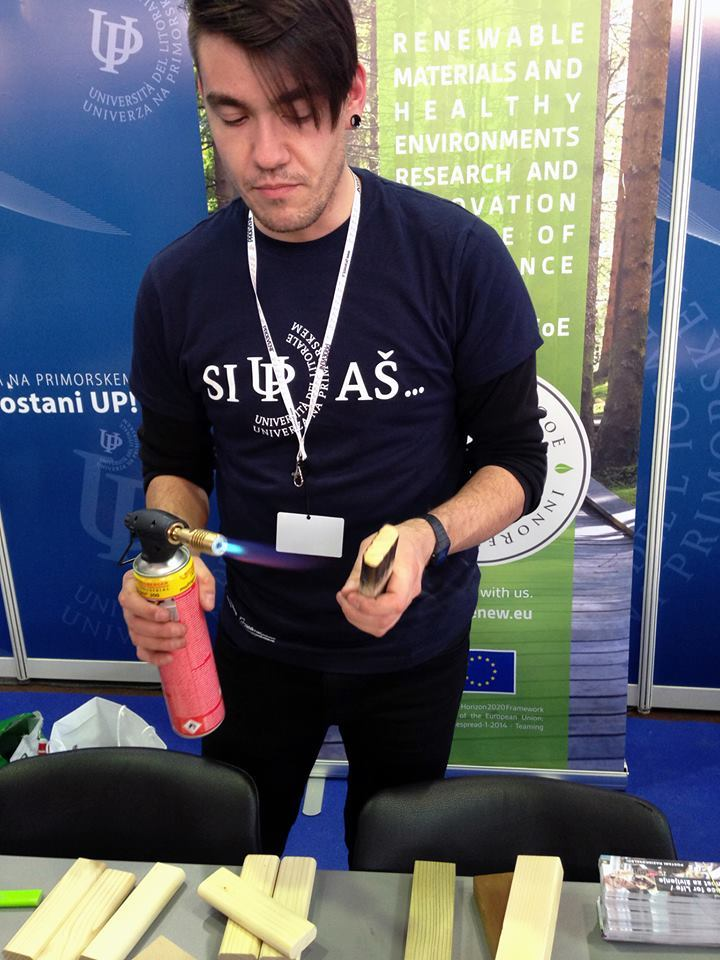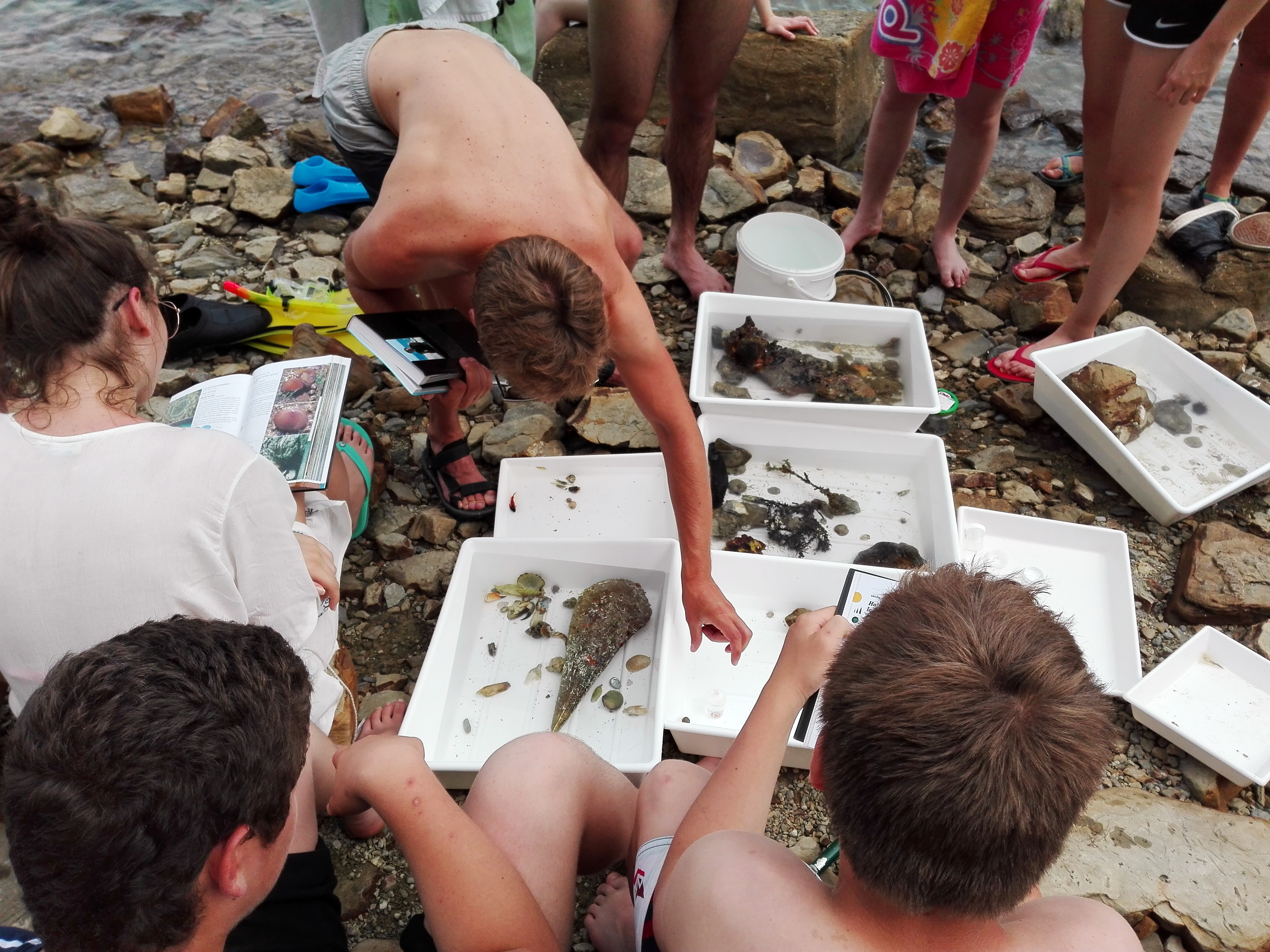SI | EN# Raziskovalni matematični seminar - Arhiv

 2021 2020 2019 2018 2017 2016 2015 2014 2013 2012 2011 2010
 1 2 3 4 5 6 7 8 9 10 11 12
Datum in ura / Date and time: 30.3.15
(10:00-11:00)
Predavalnica / Location: FAMNIT-POSTA
Predavatelj / Lecturer: Alexander Ivanov (Imperial Collage London, Faculty of Natural Sciences)
Naslov / Title: Locally and weakly locally projective actions
Vsebina / Abstract:

Let G be a subgroup in the automorphism group of a graph X. The action of G on X is said to be weakly locally projective of type (n(x),q(x)) if for every vertex x of X the stabilizer G(x) of x in G induces on the set of neighbours of x a permutation group, which contains a normal subgroup isomorphic to the special linear group in dimension n(x) over the field of q(x) elements in its natural doubly transitive action on the set of 1-dimensional subspaces of the n(x)-dimensional GF(q(x))-space. Every weakly locally projective action is clearly edge-transitive. If it is also vertex-transitive the word 'weakly' is to be deleted. The main problem is to bound the order of G(x) by a function of n(x) and q(x). It was proved by D. Goldschmidt in his ground breaking paper of 1980 that |G(x)| is at most 384 if n(x)=q(x)=2 for all x; in the vertex-transitive case it amounts to the classical result by  W.Tuitte of 1947 that |G(x)| is at most 48. For the locally projective actions the problem was attacked by R.Weiss and others before it was completely solved by V.I.Trofimov at the turn of the century. I would like to discuss locally projective subglaphs in weakly locally projective graphs as a possible path towards  reducing the problem from weakly to non-weakly projective actions in the case when q(x)=2 for some vertex x of X.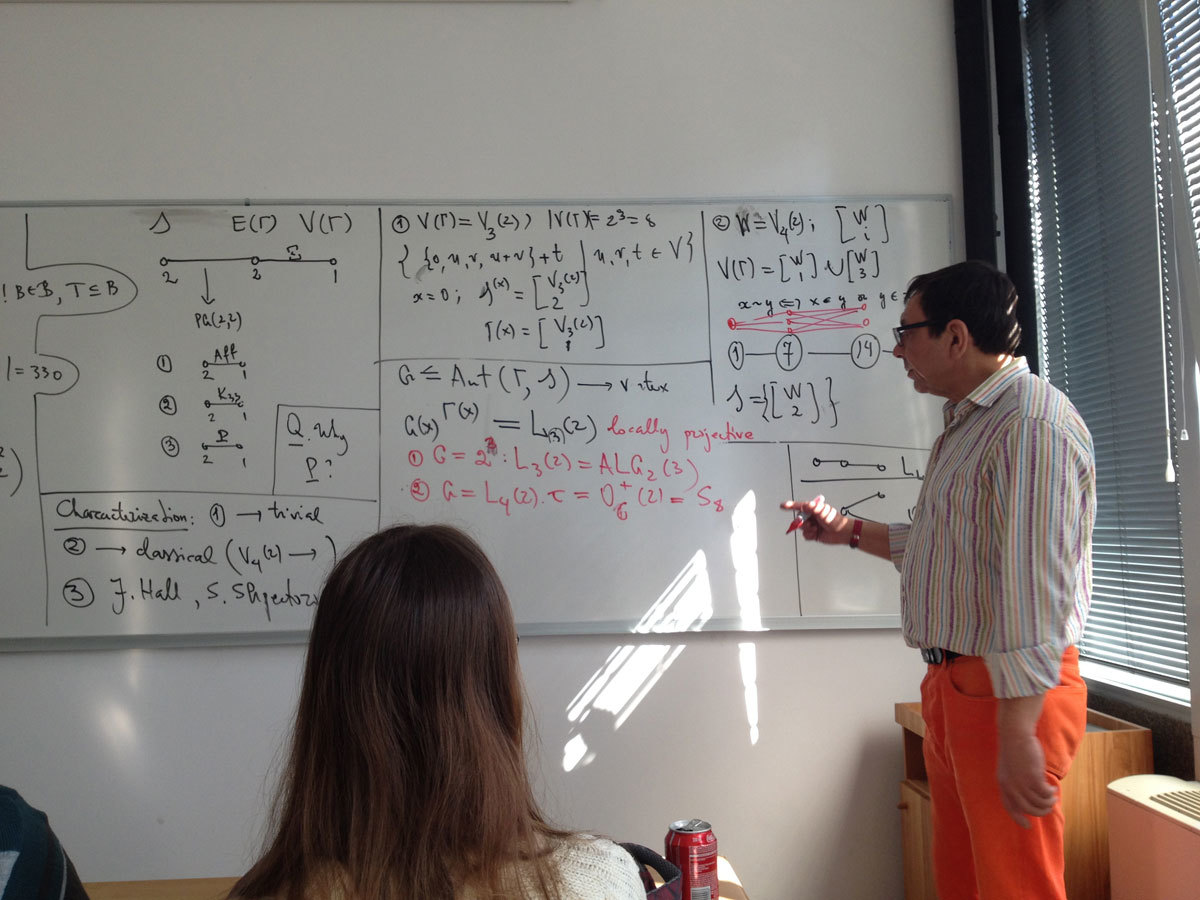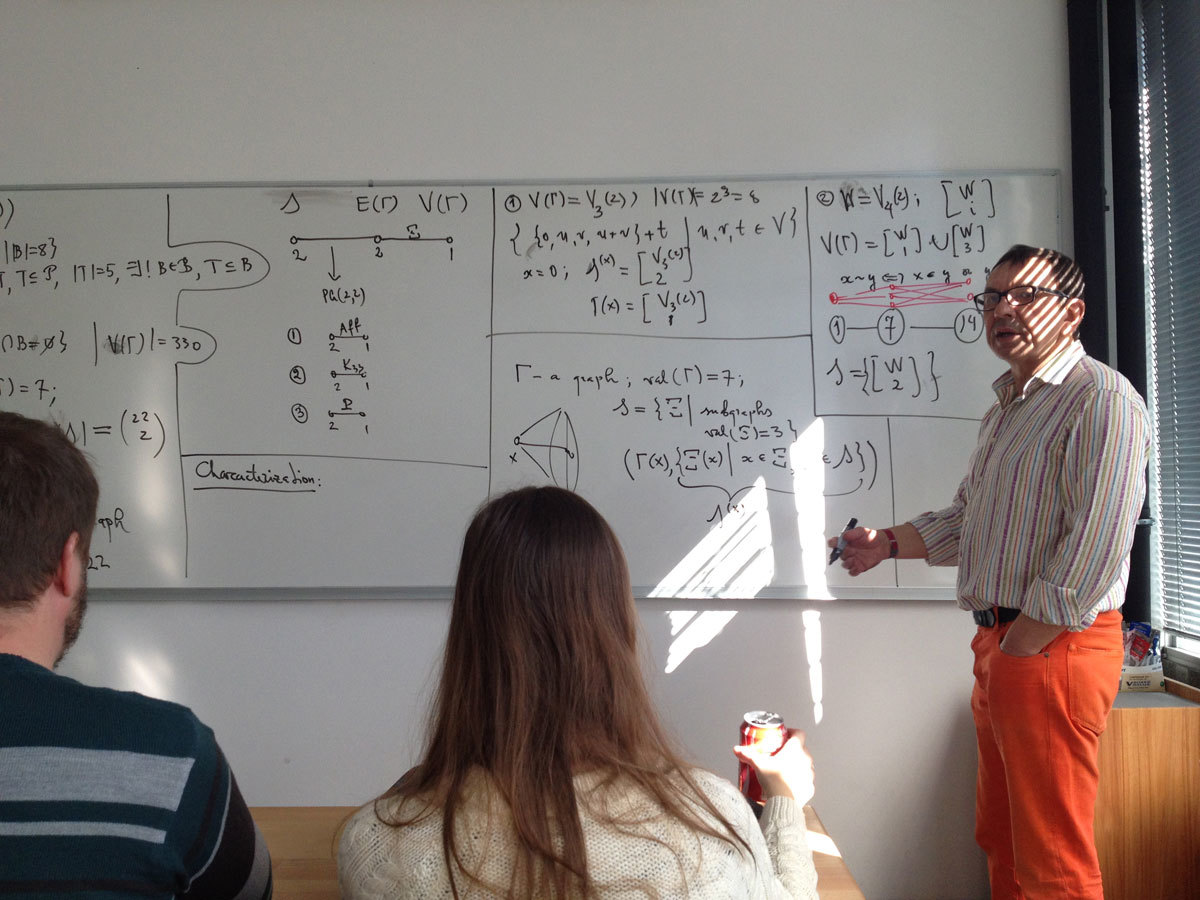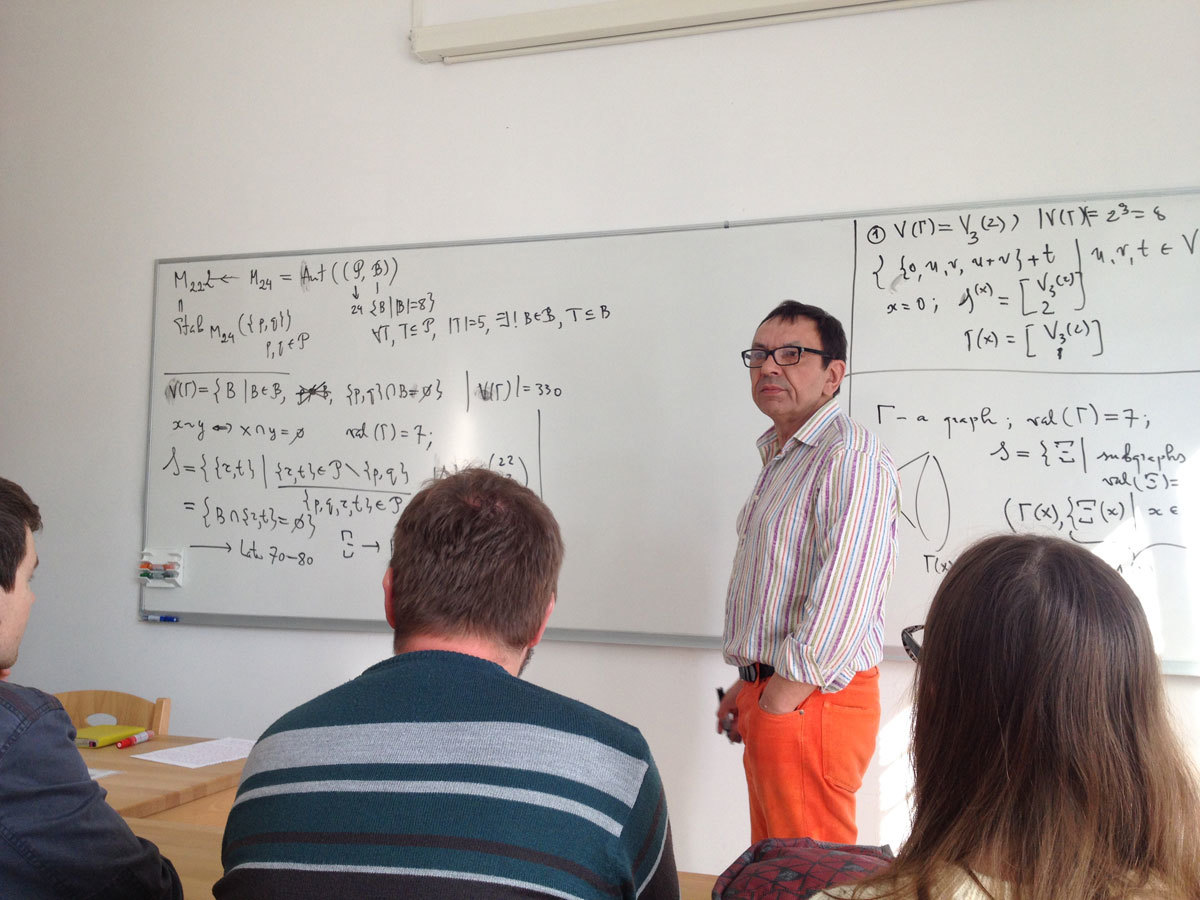Datum in ura / Date and time: 24.3.15
(14:00-15:00)
Predavalnica / Location: FAMNIT-VP
Predavatelj / Lecturer: Alexey Medvedev (Sobolev Institute of Mathematics, Novosibirsk, Russia and Central European University, Budapest, Hungary)
Naslov / Title: Hamiltonian cycles based on independent even cycles in the Pancake graph.
Vsebina / Abstract:

The Pancake graphis the Cayley graph on the symmetric group of permutations of {1,2,…,n} with the generating set of prefix-reversals. By today two Hamiltonian cycles` constructions are known: the first was given by S. Zaks in 1984 in terms of suffix-reversals,the second was suggested by A. Williams and J. Sawada in 2013 along with the general construction of greedy Prefix-reversal Gray codes.

In this talk we show that both constructions are based on the maximal sets of independent cycles. We define the family of maximal sets of independent cycles of even length in the Pancake graph, present the definition of the Hamiltonian cyclebased on independent cycles in this graph and study the existence of such Hamiltonian cycles. We also show that greedy Prefix-reversal Gray codes are characterized by our construction and provide the necessary condition of existence of these cycles.

At the end we present the open questions for the related research.

This work is joint work with Elena Konstantinova (Sobolev Institute of Mathematics and Novosibirsk State University, Novosibirsk, Russia).

Datum in ura / Date and time: 23.3.15
(10:00-11:00)
Predavalnica / Location: FAMNIT-POSTA
Predavatelj / Lecturer: Karla Ferjančič (IAM, University of Primorska)
Naslov / Title: Construction of G^3 rational motion of degree eight
Vsebina / Abstract:

In this talk we present a construction of a rigid body motion with point trajectories being rational spline curves of degree eight joining together with G^3 smoothness, which means that also the torsion is continuous.
The motion is determined through the interpolation of positions and derivative data up to order three in the geometric sense. Nonlinearity involved in a spherical part of construction results in a single univariate quartic equation which yields solutions in a closed form. We give some sufficient conditions on the regions for the curvature data, implying the existence of a real admissible solution. The algorithm how to choose appropriate data is presented too. The theoretical results are substantiated with numerical examples.

These results are joint work with Marjeta Krajnc and Vito Vitrih.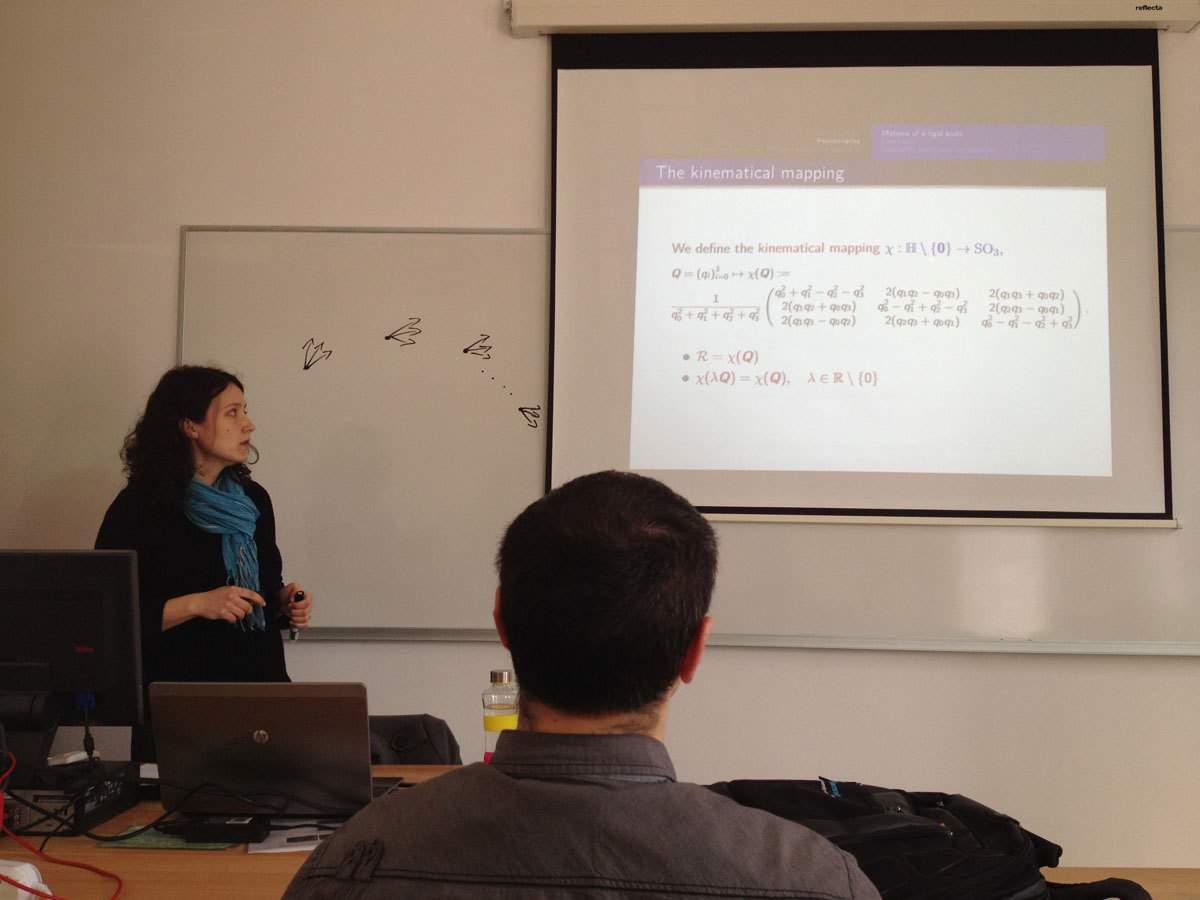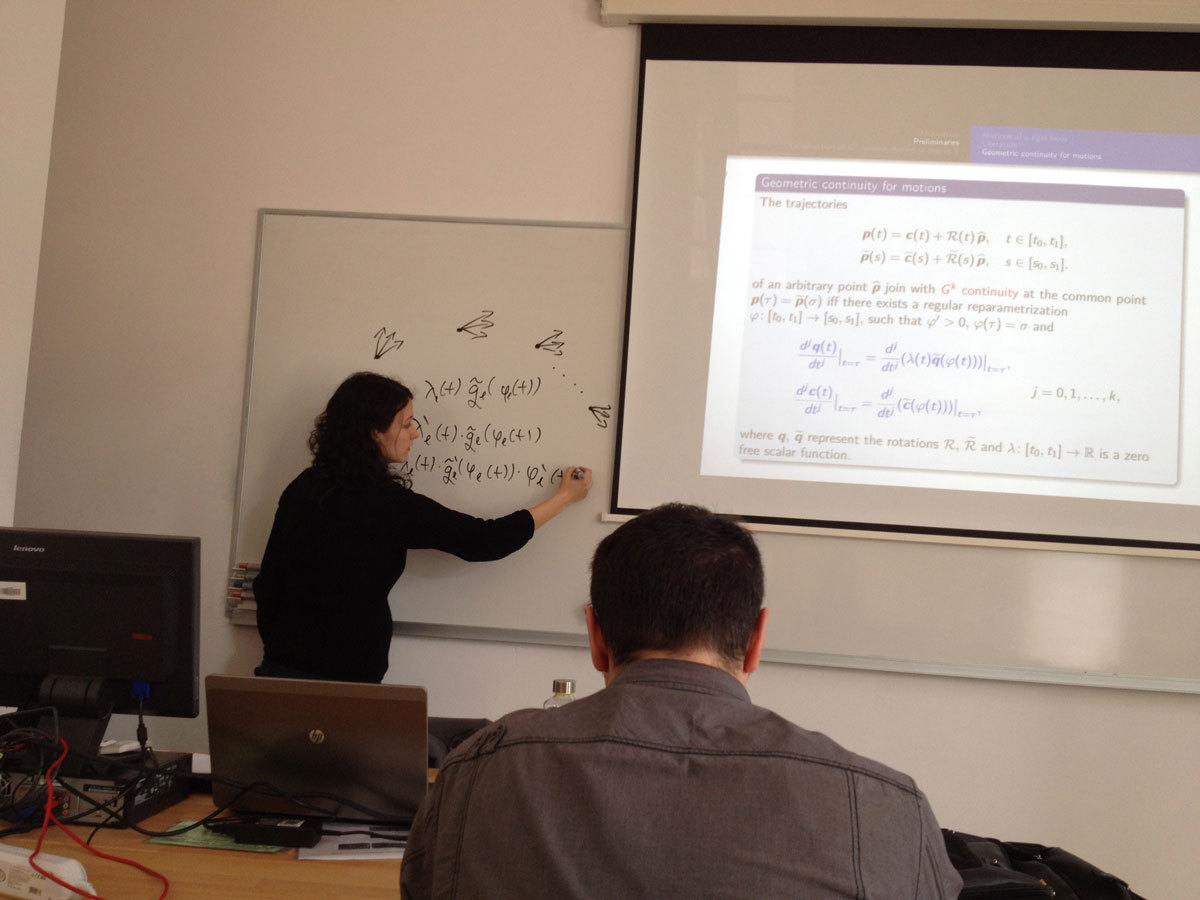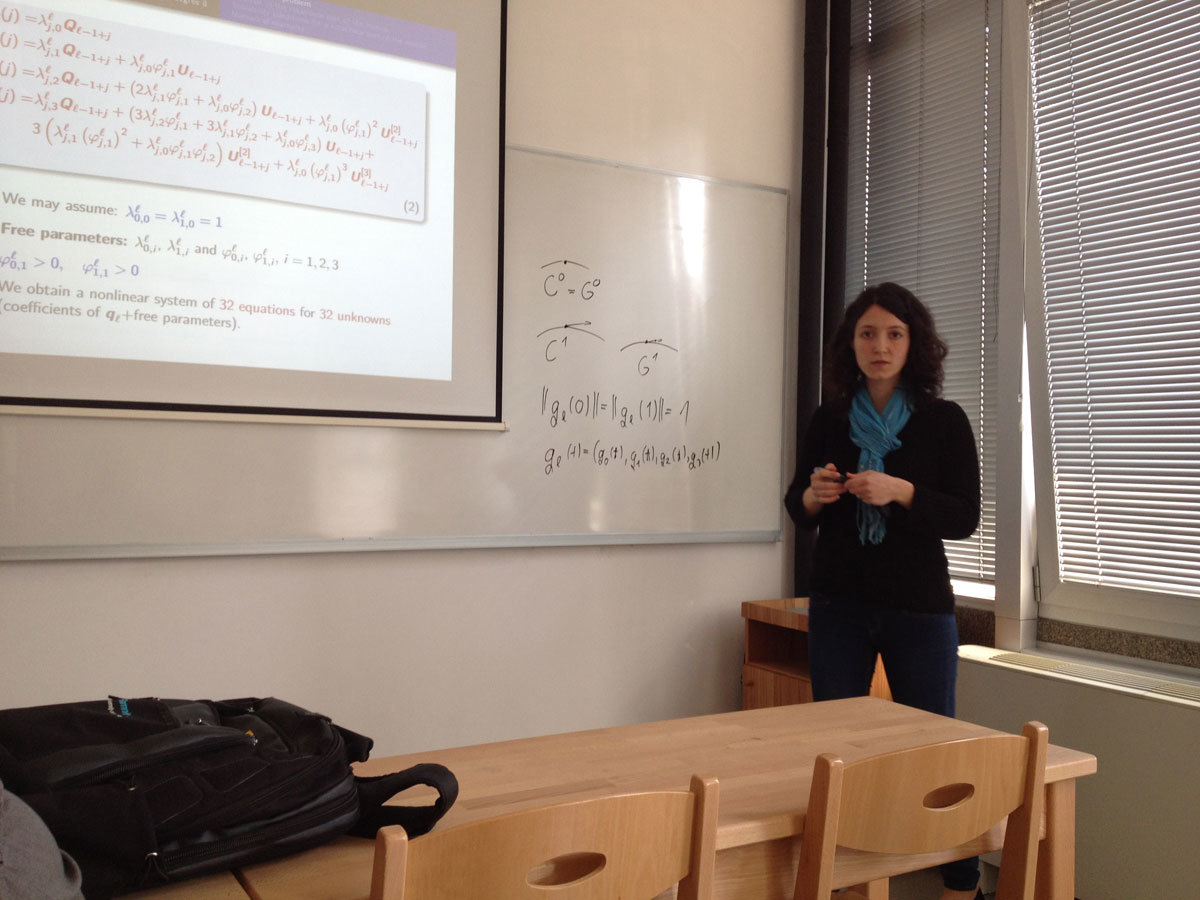Datum in ura / Date and time: 16.3.15
(10:00-11:00)
Predavalnica / Location: FAMNIT-POSTA
Predavatelj / Lecturer: Samir Hodžić (UP FAMNIT)
Naslov / Title: Generalized bent functions - some general construction methods and related necessary and sufficient conditions
Vsebina / Abstract:

In this talk we present a broader theoretical framework useful in studying the properties of so-called generalized bent functions. We give the sufficient conditions (and in many cases also necessary) for generalized bent functions when these functions are represented as a linear combination of: generalized bent; Boolean bent; and a mixture of generalized bent and Boolean bent functions. These conditions are relatively easy to satisfy and by varying the variables that specify these linear combinations many different classes of generalized bent functions can be derived. In particular, based on these results, we provide some generic construction methods of these functions and demonstrate that some previous methods are just special cases of the results given in this talk.

http://link.springer.com/article/10.1007/s12095-015-0126-9

Datum in ura / Date and time: 9.3.15
(10:00-11:00)
Predavalnica / Location: FAMNIT-POSTA
Predavatelj / Lecturer: dr. Marko Orel (UP IAM and UP FAMNIT)
Naslov / Title: Adjacency preservers on invertible hermitian matrices
Vsebina / Abstract:

Consider the graph with the vertex set formed by all $n\times n$ invertible hermitian matrices with coefficients in a finite field $\mathbb{F}_{q^2}$, where two matrices form an edge $\{A,B\}$ if and only if the rank of $A-B$ is one.

In a seminar talk about a year ago I showed that this graph is a core (the case $q=3$ was not considered), which means that all its endomorphisms are automorphisms.

In this talk I will describe the characterization of all endomorphisms (i.e. automorphisms) for $q\geq 4$.